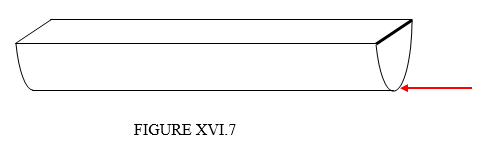$$\require{cancel}$$

16.6: Centre of Pressure

•• Contributed by Jeremy Tatum
• Emeritus Professor (Physics & Astronomy) at University of Victoria

“The centre of pressure is the point at which the pressure may be considered to act.” This is a fairly meaningless sentence, yet it is not entirely devoid of all meaning. If you refer to the left hand side of Figure XVI.5, you will see an infinite number (I’ve drawn only eight) of forces. If you were to replace all of these forces by a single force, where would you put it? Or, more precisely, if you were to replace all of these forces by a single force such that the (first) moment of this force about a line through the surface of the fluid is the same as the (first) moment of all the actual forces, where would you place this single force? You would place it at the centre of pressure. The depth of the centre of pressure is a depth such that the moment of the total force on a vertical surface about a line in the surface of the fluid is the same as the moment of all the hydrostatic forces about a line in the surface of the fluid. I shall use the Greek letter $$\zeta$$ to indicate the depth of the centre of pressure. We can continue to use Figure XVI.5.The force on the strip of area $$dA$$ at depth $$z$$ is, as we have seen, $$\rho gzdA$$, so the first moment of that force is $$\rho gz^{2}dA$$. The total moment is therefore $$\rho g\int z^{2}dA$$ which is, by definition of radius of gyration $$k$$, (see Chapter 2), $$\rho gk^{2}A$$. The total force, as we have seen, is $$pg\overline{z}A$$ and the total moment is to be this times $$\zeta$$. Thus the depth of the centre of pressure is

$\zeta = \dfrac{k^{2}}{\overline{z}} \label{16.6.1}$

Example $$\PageIndex{1}$$

A semicircular trough of radius $$a$$ is filled with water, density $$\rho$$. One semicircular end of the trough is freely hinged at its diameter (the thick line in the Figure). What force must be exerted at the bottom of the trough to prevent the end from swinging open?

Solution

The area of the semicircle is $$\frac{1}{2}\pi a^{2}$$. The depth of the centroid is $$\frac{4a}{3\pi}$$ so the total hydrostatic force is $$\frac{2}{3}\rho ga^{2}$$. The square of the radius of gyration is $$\frac{1}{4}a^{2}$$, so the depth of the centre of pressure is $$\zeta = \frac{3\pi}{16}a$$. The moment of the hydrostatic forces is therefore $$\frac{1}{8}\pi\rho ga^{3}$$. If the required force is $$F$$, this must equal $$Fa$$, and therefore $$F=\frac{1}{8}\pi\rho ga^{2}$$.